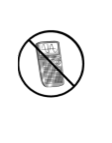### Home > CAAC > Chapter 14 > Lesson 14.2.1.6 > Problem2-89

2-89.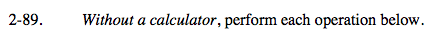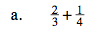$\left(\frac{4}{4}\right)\cdot\frac{2}{3}+\frac{1}{4}\cdot\left(\frac{3}{3}\right)$

$\frac{8}{12}+\frac{3}{12}$

$\frac{11}{12}$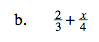Refer to part (a).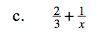$\left(\frac{x}{x}\right)\cdot\frac{2}{3}+\frac{1}{x}\cdot\left(\frac{3}{3}\right)$

$\frac{2\textit{x}}{3\textit{x}}+\frac{3}{3\textit{x}}$

$\frac{2\textit{x}+3}{3\textit{x}}$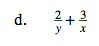Refer to part (c).